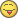# CnCNet Forums

Search In
• More options...
Find results that contain...
Find results in...

## Recommended Posts

d/dx(0.5^x) = -0.693147×0.5^x

d/dx(1^x) = 0

d/dx(1.5^x) = 0.405465×1.5^x

d/dx(2^x) = 2^x log(2)

d/dx(2.71^x) = 0.996949×2.71^x

d/dx(e^x) = e^x

d/dx(2.72^x) = 1.00063×2.72^x

d/dx(3.14^x) = 1.14422×3.14^x

d/dx(pi^x) = pi^x log(pi)

Explain, anyone?##### Share on other sites

d/dx(0.5^x) = -0.693147×0.5^x

d/dx(1^x) = 0

d/dx(1.5^x) = 0.405465×1.5^x

d/dx(2^x) = 2^x log(2)

d/dx(2.71^x) = 0.996949×2.71^x

d/dx(e^x) = e^x

d/dx(2.72^x) = 1.00063×2.72^x

d/dx(3.14^x) = 1.14422×3.14^x

d/dx(pi^x) = pi^x log(pi)

Explain, anyone?Magic.

##### Share on other sites

d/dx(0.5^x) = -0.693147×0.5^x

d/dx(1^x) = 0

d/dx(1.5^x) = 0.405465×1.5^x

d/dx(2^x) = 2^x log(2)

d/dx(2.71^x) = 0.996949×2.71^x

d/dx(e^x) = e^x

d/dx(2.72^x) = 1.00063×2.72^x

d/dx(3.14^x) = 1.14422×3.14^x

d/dx(pi^x) = pi^x log(pi)

Explain, anyone?Do it by definition if it confuses you...##### Share on other sites

The derivative of a function gives its instantaneous rate of change, or slope, at any given point. The exponential function just so happens to have the same value as its slope at every point. The simplest example is exp^0=1, where the slope of exp^x at x=0 is also 1. Continue inductively from there.

##### Share on other sites

Strategst, I know what a derivative is.

Magic.

Do it by definition if it confuses you...These are the best answers. Except, I'm not sure I can simplify the limit without L'H and knowing that the derivative of e^x is e^x.##### Share on other sites

These are the best answers. Except, I'm not sure I can simplify the limit without L'H and knowing that the derivative of e^x is e^x.There is something called a Google search, which gives the answer (without the L'Hospital rule)...

##### Share on other sites

Thank you, Plokite_Wolf! It is all over Google, indeed. But your link is exactly what I was looking for!## Create an account

Register a new account